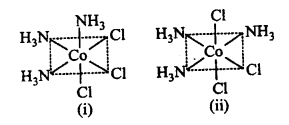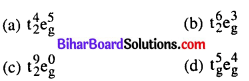# Bihar Board 12th Chemistry Objective Answers Chapter 9 Coordination Compounds

Bihar Board 12th Chemistry Objective Questions and Answers

## Bihar Board 12th Chemistry Objective Answers Chapter 9 Coordination Compounds

Question 1.
Copper sulphate dissolves in ammonia due to the formation of
(a) Cu2O
(b) [Cu(NH3)4]SO4
(c) [Cu(NH3)4]OH
(d) [Cu(H2O)4]SO4
(b) [Cu(NH3)4]SO4

Question 2.
The number of ions given by [Pt(NH3)6]Cl4 inaqueous solution will be
(a) two
(b) three
(c) five
(d) eleven
(c) fiveQuestion 3.
When one mole of each of the following complexes is treated with excess of AgNO3, which will give maximum amount of AgCl ?
(a) [Co(NH3)6]Cl3
(b)[Co(NH3)5CI]Cl2
(c) [Co(NH3)4Cl2]Cl
(d) [Co(NH3)3Cl3]
(a) [Co(NH3)6]Cl3

Question 4.
According to Werner’s theory of coordination compounds.
(a) primary valency is ionisable
(b) secondary valency is ionisable
(c) primary and secondary valencies are ionisable
(d) neither primary nor secondary valency is ionisable
(a) primary valency is ionisable

Question 5.
Which of the following primary and secondary valencies are not correctly marked against the compounds ?
(a) [Cr(NH3)6]Cl3, p = 3,s = 6
(b) K2[Pt(Cl4], p = 2, s = 4
(c) [Pt(NH3)2CI2], p = 2, s = 4
(d) [Cu(NH3)4]SO4 , p = 4, s = 4
(d) [Cu(NH3)4]SO4 , p = 4, s = 4

Question 6.
The ligand N(CH2CH2NH2)3 is
(a) bidentate
(b) tridentate

Question 7.
Which of the following is a tridentate ligand ?
(a)EDTA4-
(b) (COO)2
(c) dien
(d) NO2
(c) dienQuestion 8.
Among the following, which are ambidentate ligands ?
(i)SCN
(ii) NO
(iii) NO;
(iv) C2O4
(a) (i) and (iii)
(b) (i) and (iv)
(c) (ii) and (iii)
(d) (ii) and (iv)
(a) (i) and (iii)

Question 9.
Which of the following ligands form a chelate ?
(a) Acetate
(b) Oxalate
(c) Cyanide
(d) Ammonia
(b) Oxalate

Question 10.
Which of the following is not a neutral ligand ?
(a) H2O
(b) NH3
(c) ONO
(d) CO
(c) ONOQuestion 11.
Which of the following ligands will not show chelation ?
(a) EDTA
(b) DMG
(c) Ethane – 1, 2-diamine
(d) SCN
(d) SCN

Question 12.
The coordination number and the oxidation state of the  element E in the complex [E(en)2(C2O4)]NO2 (where (en) is ethylenediamine) are, respectively
(a) 6 and 3
(b) 6 and 2
(c) 4 and 2
(d) 4 and 3
(a) 6 and 3

Question 13.
The correct IUPAC name of the coordination compound K3[Fe(CN)5NO] is
(a) potassium pentacyanonitrosylferrate (II)
(b) potassium pentacyanonitroferrate(III)
(c) potassium nitritopentacyanoeferrate (IV)
(d) potassium nitritepentacyanoiron (II)
(a) potassium pentacyanonitrosylferrate (II)

Question 14.
Correct formula of tetraamminechloronitroplatinum (IV) sulphate can be written as
(a) [ Pt(NH3 )4 (ONO)Cl ]SO4
(b) [Pt(NH3)4Cl2NO2]2SO4
(c) [Pt(NH3)4(NO2)Cl]SO4
(d) [PtCl (ONO) NH3(SO4)]
(c) [Pt(NH3)4(NO2)Cl]SO4

Question 15.
Which among the following will be named as dibromidobis (ethylenediamine) chromium (III) bromide ?
(a) [Cr(en)2Br2]Br
(b) [Cr(en)Br]
(c) [Cr(en)Br2]Br
(d) [Cr(en)3]Br3
(a) [Cr(en)2Br2]BrQuestion 16.
The name of the compound [Co(NH3)5NO2]Cl2 will be
(a) pentaamminonitrocobalt (II) chloride
(b) pentaamminenitrochloridecobaltate (III)
(c) pentaamminenitrocobalt (III) chloride
(d) pentanitrosoamminechlorocobaltate (III)
(c) pentaamminenitrocobalt (III) chloride

Question 17.
How many geometrical isomers are there for [Co(NH3)2Cl4] (octahedral) and [AuCl2Br2] (square planar) ?
(a) Two cis and trans, no geometrical isomers
(b) Two cis and trans, two cis and trans
(c) No geometrical isomers, two cis and trans
(d) No geometrical isomers, no geometrical isomers
(b) Two cis and trans, two cis and trans

Question 18.
Which of the following will not show geometrical isomerism ?
(a) [Cr(NH3)4Cl2]Cl
(b) [Co(en)2Cl2]CI
(c) [Co(NH3)5NO2]Cl2
(d) [Pt(NH3)2Cl2]
(c) [Co(NH3)5NO2]Cl2Question 19.
Which of the following shows maximum number of isomers ?
(a) [Co(NH3)4Cl2]
(b) [Ni(en)(NH3)4]2+
(c) [Ni(C2O4)(en)2]2-
(d) [Cr(SCN)2(NH3)4]+
(d) [Cr(SCN)2(NH3)4]+

Question 20.
Which of the following complexes exists as pair of enantiomers ?
(a) [Co(NH3)4Cl2]+
(b) [Cr(en)3]3+
(c) [Co(P(C2H5)3)2ClBr]
(d) trans- [Co(en)2Cl2 ]+
(b) [Cr(en)3]3+

Question 21.
Two isomers of a compound Co(NH3)3Cl3(MA3B3type) are shown in the figures.The isomers can be classified as
(a) (i) fac-isomers (ii) mer-isomer
(b) (i) optical-isomer (ii) trans-isomer
(c) (i) mer-isomer (ii) fac-isomer
(d) (i) trans-isomer (ii) cis-isomer
(a) (i) fac-isomers (ii) mer-isomer

Question 22.
Which of the following compounds exhibits linkage isomerism ?
(a) [Co(en)3]Cl3
(b) [Co(NH3)6][Cr(en)3]
(c) [Co(en)2 (NO2)Cl]Br
(d) [Co(NH3)5Cl]Br2
(c) [Co(en)2 (NO2)Cl]Br

Question 23.
[Pt(NH3)4][CuCl4] and tCu(NH3)4][PtCl4] are known is
(a) ionisation isomers
(b) coordination isomers
(d) polymerisation isomers
(b) coordination isomersQuestion 24.
Which of the following isomers will give white precipitate with BaCl2 solution ?
(a) [Co(NH3)5SO4]Br
(b) [Co(NH3)5 Br]SO4
(c) [Co(NH3)4(SO4)2]Br
(d) [Co(NH3)4 Br(SO4)]
(b) [Co(NH3)5 Br]SO4

Question 25.
CrCl3.6H2O exists in different isomeric forms which show different colours like violet and green. This is due to
(a) ionisation isomerism
(b) coordination isomerism
(c) optical isomerism
(d) hydrate isomerism
(d) hydrate isomerism

Question 26.
The hybridisation involved in [Co(C2O4)3]3- is
(a) sp3d2
(b) sp3d3
(c) dsp3
(d) d2sp3
(d) d2sp3

Question 27.
Which of the following complexes will have tetrahedral shape ?
(a) [PdCl4]2-
(b) [Pd(CN)4]2-
(c) [Ni(CN)4l2-
(d) [NiCl4]2-
(d) [NiCl4]2-Question 28.
The complex ion which has no d-electrons in the central metal atom is
(a) [MnO4]
(b) [Co(NH3)6]3+
(c) [Fe(CN)6]3-
(d) [Cr(H2O)6]+
(a) [MnO4]

Question 29.
The lowest value paramagnetism is shown by
(a) [Co(CN)6]3-
(b) [Fe(CN)6]3-
(c) [Cr(CN)6]3-
(d) [Mn(CN)6]3-
(a) [Co(CN)6]3-

Question 30.
[CoF6]3- is
(a) paramagnetic and undergoes sp3d2 hybridisation
(b) diamagnetic and undergoes d2sp3 hybridisation
(c) paramagnetic and undergoes sp3d hybridisation
(d) diamagnetic and undergoes sp3 hybridisation
(a) paramagnetic and undergoes sp3d2 hybridisationQuestion 31.
The magnitude of magnetic moment (spin only) of [NiCl4]2- will be
(a) 2.82B.M.
(b) 3.25B.M.
(c) 1.23 B.M.
(d) 5.64 B.M.
(a) 2.82B.M.

Question 32.
Which of the following has largest paramagnetism ?
(a) [Cr(H2O)6]3+
(b) [Fe(H2O)6]2+
(c) [Cu(H2O)6]2+
(d) [Zn(H2O)2]2+
(b) [Fe(H2O)6]2+

Question 33.
Which of the following descriptions about [FeCl6 ]4- is correct about the complex ion ?
(a) sp3 d, inner orbital complex, diamagnetic
(b) sp3 d2, outer orbital complex, paramagnetic
(c) d2sp3, inner orbital complex, paramagnetic
(d) d2sp3, outer orbital complex, diamagnetic
(b) sp3 d2, outer orbital complex, paramagnetic

Question 34.
When excess of ammonia is added to copper sulphate solution, the deep blue coloured complex is formed. The complex is
(a) tetrahedral and paramagnetic
(b) tetrahedral and diamagnetic
(c) square planar and diamagnetic
(d) square planar and paramagnetic
(d) square planar and paramagneticQuestion 35.
Among the following compounds which is both paramagnetic and coloured ?
(a) K2Cr2O7
(b) [Co(SO4)]
(c) (NH4)2[TiCl6]
(d) K3[Cu(CN)4]
(b) [Co(SO4)]

Question 36.
The spin only magnetic moment value of Cr(CO)6 is
(a) 2.84 B.M.
(b) 4.90 B.M.
(c) 5.92 B.M.
(d) O.B.M.
(d) O.B.M.

Question 37.
Which of the following complexes will show maximum paramagnetism ?
(a) 3d4
(b) 3d5
(c) 3d6
(d) 3d7
(b) 3d5

Question 38.
Electronic configuration of [Cu(NH3)6]2+ on the basis of crystal field splitting theory is(b)

Question 39.
Which of the following shall form an octahedral complex ?
(a) d4 (low spin)
(b) d8 (high spin)
(c) d6 (low spin)
(d) None of these
(c) d6 (low spin)

Question 40.
The value of the ‘spin only’ magnetic moment for one of the following configuration is 2.84 BM. The correct one is
(a) d4(in strong ligand field)
(b) d4 (in weak ligand field)
(c) d3 (in weak as well as in strong fields)
(d) d5 (in strong ligand field)
(a) d4(in strong ligand field)Question 41.
CuSO4.5H2O is blue in colour while CuSO4 is colourless due to
(a) presence of strong field ligand in CuSO4.5H2O
(b) due to absence of water (ligand), d-d transitions are not possible in CuSO4
(c) anhydrous CuSO4 undergoes d-d transitions due to crystal field splitting
(d) colour is lost due to loss of unpaired electrons
(b) due to absence of water (ligand), d-d transitions are not possible in CuSO4

Question 42.
[Fe(CN)6]4- and [Fe(H2O)6]2+ show different colours in dilute solution because
(a) CN is a strong field ligand and H2O is a weak field ligand hence magnitude of CFSE is different
(b) both CN and H2O absorb same wavelength of energy
(c) complexes of weak field ligands are generally colourless
(d) the sizes of CN and H2O are different hence their colours are also different
(d) the sizes of CN and H2O are different hence their colours are also different

Question 43.
The terminal and bridged CO ligands in the compound [Co2(CO)8] are respectively
(a) 0, 2
(b) 6, 1
(c) 5,2
(d) 6, 2
(b) 6, 1Question 44.
The geometry possessed by [Ni(CO)4] is
(a) tetrahedral
(b) square planar
(c) linear
(d) octahedral
(a) tetrahedral

Question 45.
Cr-C bond in the compound [Cr(CO)6] shows 71- character due to
(a) covalent bonding
(b) coordinate bonding
(c) synergic bonding
(d) ionic bonding
(c) synergic bondingQuestion 46.
The overall complex dissociation equilibrium constant for the complex [Cu(NH3)4]2+ ion will be (P4 for this complex is 2.1 x 1013)
(a) 7 x 10-14
(b) 2.1 x 1013
(c) 11.9 x 10-2
(d) 2.1 x 103
(a) 7 x 10-14

Question 47.
Mark the incorrect match
(a) Insulin – Zinc
(b) Haemoglobin – Iron
(c) Vitamin Bp –  Cobalt
(d) Chlorophyll – Chromium
(d) Chlorophyll – Chromium

Question 48.
The correct IUPAC name of [Pt(NH3)2Cl2] is ……………….
(a) diamminedichloridoplatinum (II)
(b) diamminedichloridoplatinum (IV)
(c) diamminedichloridoplatinum (0)
(d) dichloridodiammineplatinum (IV)
(a) diamminedichloridoplatinum (II)

Question 49.
The stabilisation of coordination compounds due to chelation is called the chelate effect. Which of the following is the most stable complex species ?
(a) [Fe(CO)5]
(b) [Fe(CN)6]-
(c) [Fe(C2O4)3]3-
(d) [Fe(H2O)6]3+
(c) [Fe(C2O4)3]3-

Question 50.
Indicate the complex ion which shows geometrical isomerism.
(a) [Cr(H2O)4Cl2]+
(b) [Pt(NH3)3Cl]
(c) [Co(NH3)6]3+
(d) [Co(CN)5(NC)]3-
(a) [Cr(H2O)4Cl2]+Question 51.
The CFSE for octa hedral [CoCl6]4- is 18,000 cm-1. The CFSE for tetrahedral [CoCl4 ]2 will be
(a) 18,0000 cm-1
(b) 16,000 cm-1
(c) 8,000 cm-1
(d) 20,000 cm-1
(c) 8,000 cm-1

Question 52.
The compounds [Co(SO4)(NH3)s] Br and [Co(SO4)(NH3)5]Cl represent
(b) ionisation isomerism
(c) coordination isomerism
(d) no isomerism
(d) no isomerism

Question 53.
A chelating agent has two or more than two donor atoms to bind to a single metal ion. Which of the following is not a chelating agent ?
(a) Thiosulphato
(b) Oxalato
(c) Glycinato
(d) Ethane-1. 2-diamine
(a) Thiosulphato

Question 54.
Which of the following species is not expected to be a ligand ?
(a) NO
(b) NH4
(c) NH2CH2CH2NH2
(d) CO